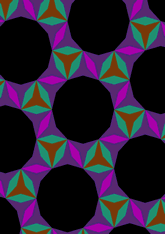# Derived from 3**4.6 with digons added, angle 30 degreesdata145/D346B

## Geometry

• The symmetry group of the tiling is 632 (p6).
• All the internal angles of the constituent polygons are a multiple of 30°.
• Contains two regular two-pointed star polygons with vertex angle of 30°.
• Contains two regular three-pointed star polygons with vertex angle of 30°.
• Contains one regular 12-gon.
• The tiling satisfies the two-colour condition.
• The tiling is edge-to-edge.
• As drawn, contains about 197 polygons.

## References

Publications referenced:
1. Derived from figure on page 63 of B GrÃ¼nbaum and G C Shephard. Tilings and Patterns, W H Freeman, 1987. ISBN 071671193. [gands] {Most important work in this area.}
2. Uniform Star Polygons with Digons of B A Wichmann. The World of Patterns, World Scientific, 2001. ISBN 981024619. [wich2] {Only new patterns ascribed to this.}

v53